# Lisp and scheme i

12 de May de 2016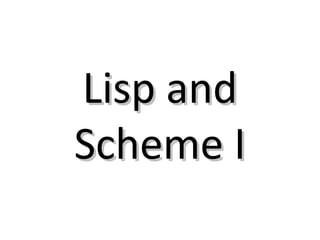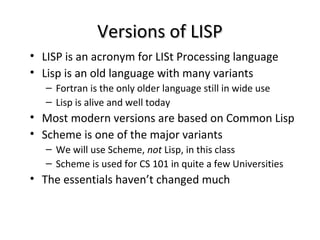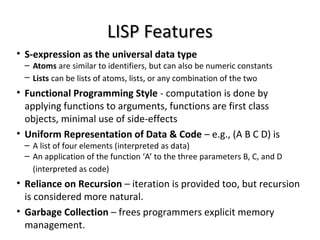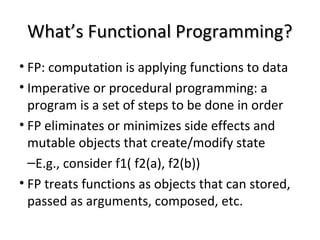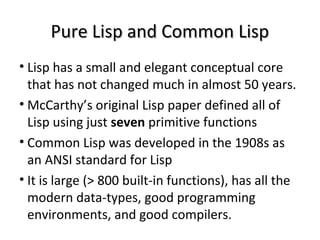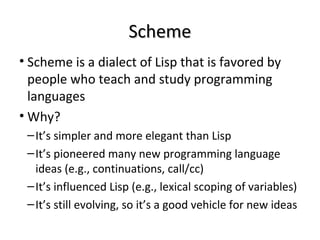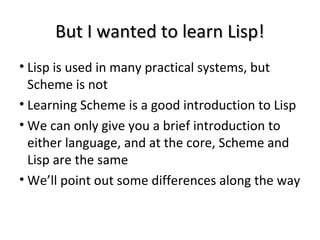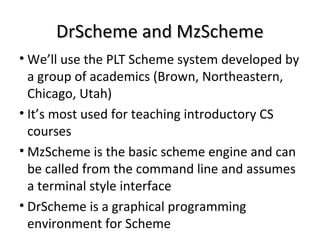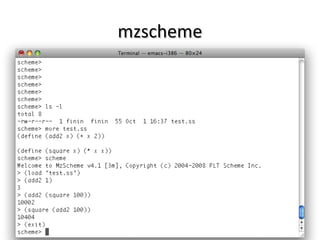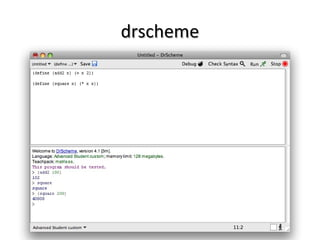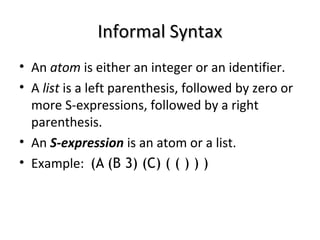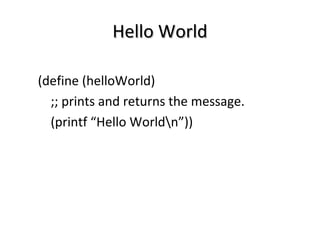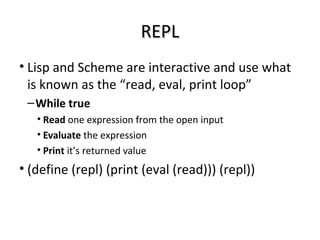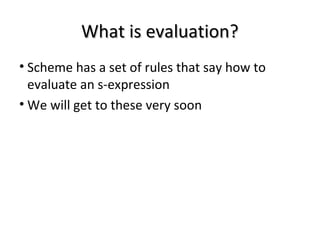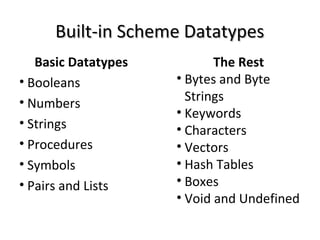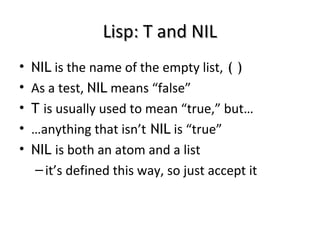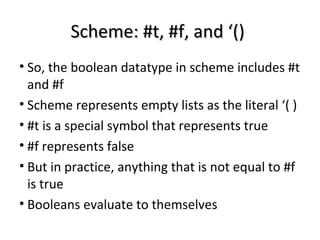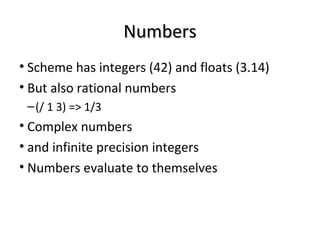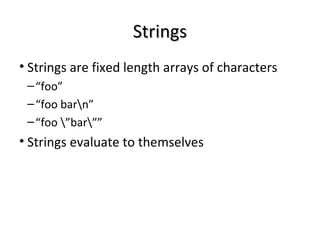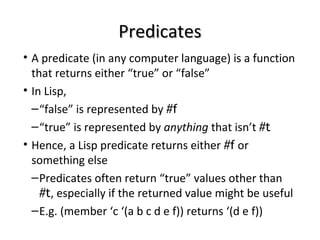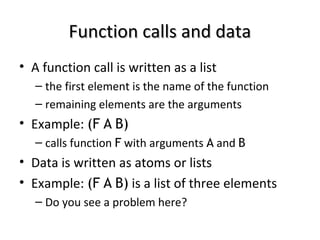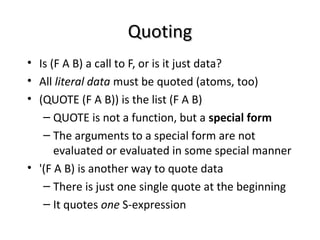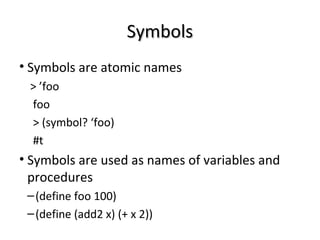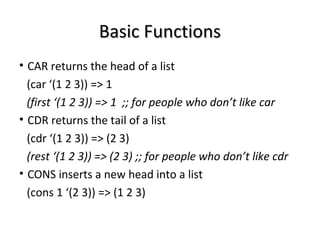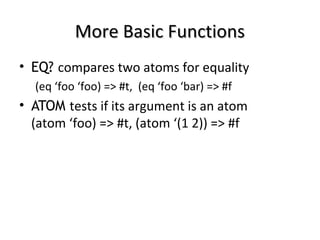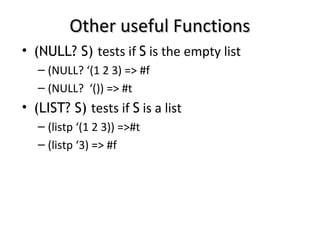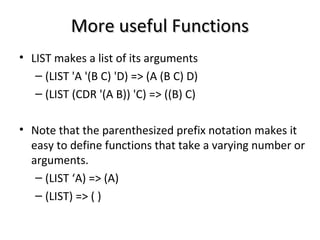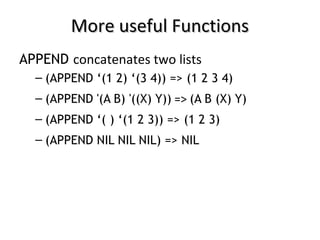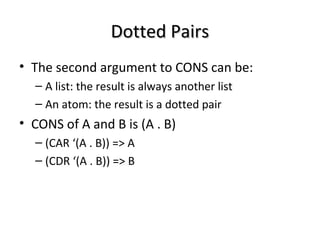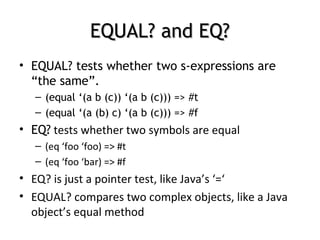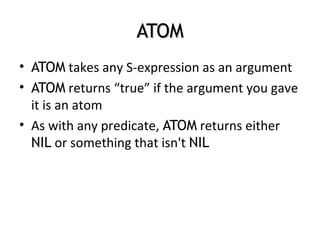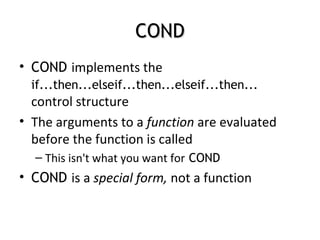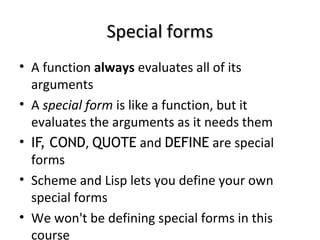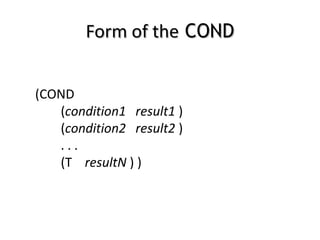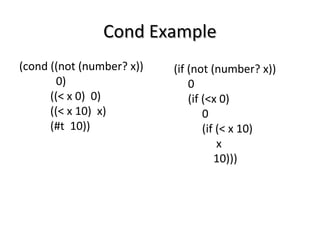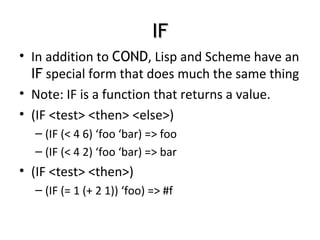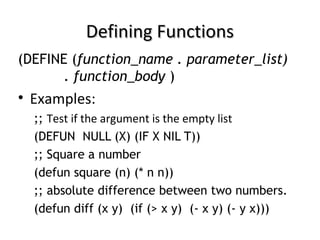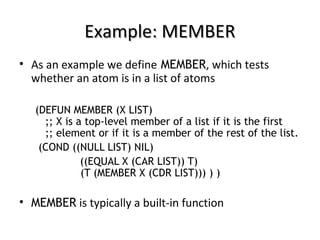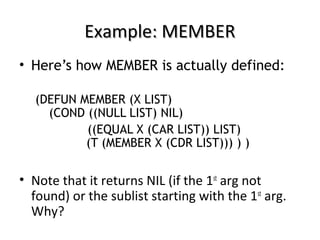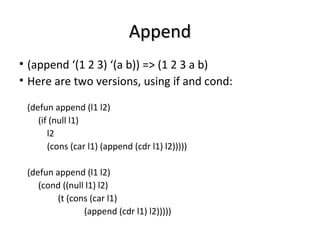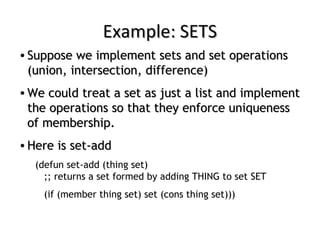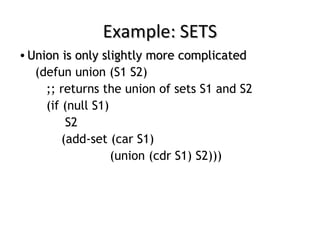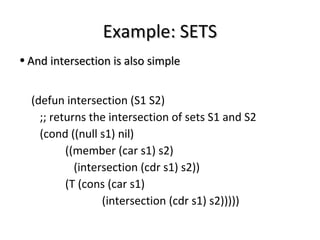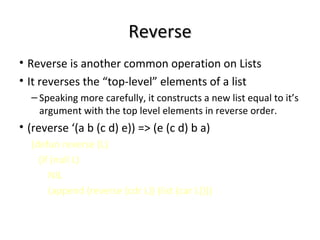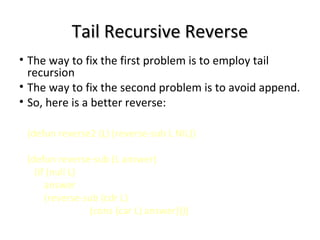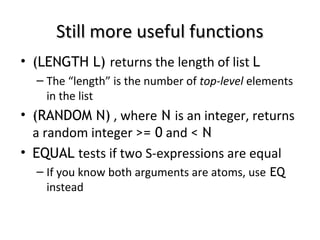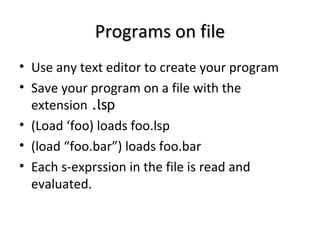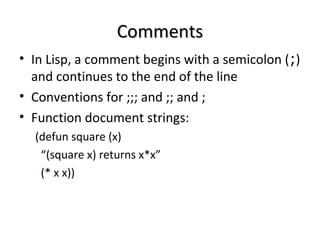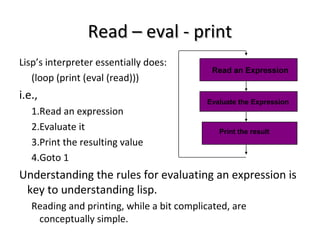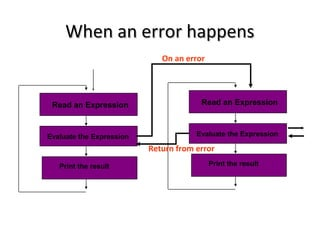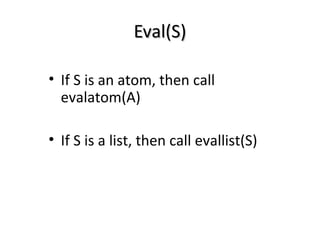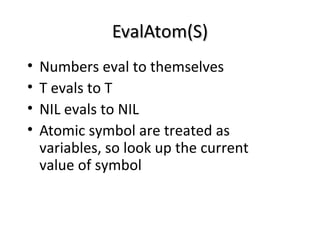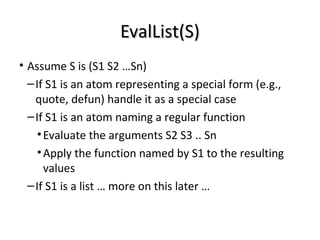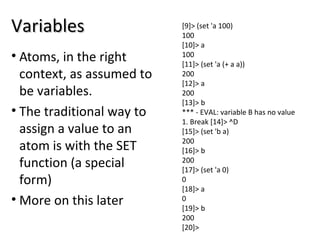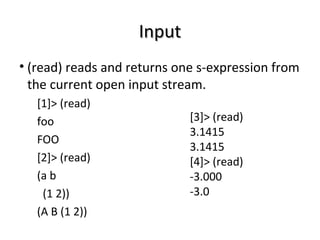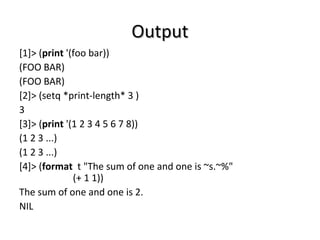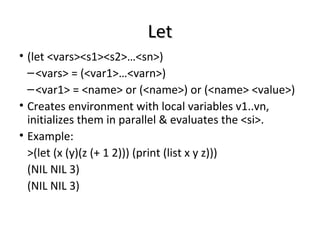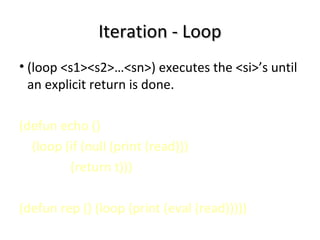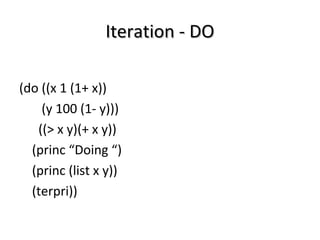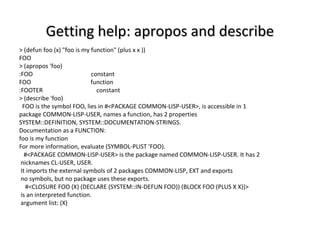1 de 62

### Lisp and scheme i

• 2. Versions of LISPVersions of LISP • LISP is an acronym for LISt Processing language • Lisp is an old language with many variants – Fortran is the only older language still in wide use – Lisp is alive and well today • Most modern versions are based on Common Lisp • Scheme is one of the major variants – We will use Scheme, not Lisp, in this class – Scheme is used for CS 101 in quite a few Universities • The essentials haven’t changed much
• 3. LISP FeaturesLISP Features • S-expression as the universal data type – Atoms are similar to identifiers, but can also be numeric constants – Lists can be lists of atoms, lists, or any combination of the two • Functional Programming Style - computation is done by applying functions to arguments, functions are first class objects, minimal use of side-effects • Uniform Representation of Data & Code – e.g., (A B C D) is – A list of four elements (interpreted as data) – An application of the function ‘A’ to the three parameters B, C, and D (interpreted as code) • Reliance on Recursion – iteration is provided too, but recursion is considered more natural. • Garbage Collection – frees programmers explicit memory management.
• 4. What’s Functional Programming?What’s Functional Programming? • FP: computation is applying functions to data • Imperative or procedural programming: a program is a set of steps to be done in order • FP eliminates or minimizes side effects and mutable objects that create/modify state –E.g., consider f1( f2(a), f2(b)) • FP treats functions as objects that can stored, passed as arguments, composed, etc.
• 5. Pure Lisp and Common LispPure Lisp and Common Lisp • Lisp has a small and elegant conceptual core that has not changed much in almost 50 years. • McCarthy’s original Lisp paper defined all of Lisp using just seven primitive functions • Common Lisp was developed in the 1908s as an ANSI standard for Lisp • It is large (> 800 built-in functions), has all the modern data-types, good programming environments, and good compilers.
• 6. SchemeScheme • Scheme is a dialect of Lisp that is favored by people who teach and study programming languages • Why? –It’s simpler and more elegant than Lisp –It’s pioneered many new programming language ideas (e.g., continuations, call/cc) –It’s influenced Lisp (e.g., lexical scoping of variables) –It’s still evolving, so it’s a good vehicle for new ideas
• 7. But I wanted to learn Lisp!But I wanted to learn Lisp! • Lisp is used in many practical systems, but Scheme is not • Learning Scheme is a good introduction to Lisp • We can only give you a brief introduction to either language, and at the core, Scheme and Lisp are the same • We’ll point out some differences along the way
• 8. DrScheme and MzSchemeDrScheme and MzScheme • We’ll use the PLT Scheme system developed by a group of academics (Brown, Northeastern, Chicago, Utah) • It’s most used for teaching introductory CS courses • MzScheme is the basic scheme engine and can be called from the command line and assumes a terminal style interface • DrScheme is a graphical programming environment for Scheme
• 11. Informal SyntaxInformal Syntax • An atom is either an integer or an identifier. • A list is a left parenthesis, followed by zero or more S-expressions, followed by a right parenthesis. • An S-expression is an atom or a list. • Example: (A (B 3) (C) ( ( ) ) )
• 12. Hello WorldHello World (define (helloWorld) ;; prints and returns the message. (printf “Hello Worldn”))
• 13. REPLREPL • Lisp and Scheme are interactive and use what is known as the “read, eval, print loop” –While true • Read one expression from the open input • Evaluate the expression • Print it’s returned value • (define (repl) (print (eval (read))) (repl))
• 14. What is evaluation?What is evaluation? • Scheme has a set of rules that say how to evaluate an s-expression • We will get to these very soon
• 15. Built-in Scheme DatatypesBuilt-in Scheme Datatypes Basic Datatypes • Booleans • Numbers • Strings • Procedures • Symbols • Pairs and Lists The Rest • Bytes and Byte Strings • Keywords • Characters • Vectors • Hash Tables • Boxes • Void and Undefined
• 16. Lisp: T and NILLisp: T and NIL • NIL is the name of the empty list, ( ) • As a test, NIL means “false” • T is usually used to mean “true,” but… • …anything that isn’t NIL is “true” • NIL is both an atom and a list –it’s defined this way, so just accept it
• 17. Scheme: #t, #f, and ‘()Scheme: #t, #f, and ‘() • So, the boolean datatype in scheme includes #t and #f • Scheme represents empty lists as the literal ‘( ) • #t is a special symbol that represents true • #f represents false • But in practice, anything that is not equal to #f is true • Booleans evaluate to themselves
• 18. NumbersNumbers • Scheme has integers (42) and floats (3.14) • But also rational numbers –(/ 1 3) => 1/3 • Complex numbers • and infinite precision integers • Numbers evaluate to themselves
• 19. StringsStrings • Strings are fixed length arrays of characters –“foo” –“foo barn” –“foo ”bar”” • Strings evaluate to themselves
• 20. PredicatesPredicates • A predicate (in any computer language) is a function that returns either “true” or “false” • In Lisp, –“false” is represented by #f –“true” is represented by anything that isn’t #t • Hence, a Lisp predicate returns either #f or something else –Predicates often return “true” values other than #t, especially if the returned value might be useful –E.g. (member ‘c ‘(a b c d e f)) returns ‘(d e f))
• 21. Function calls and dataFunction calls and data • A function call is written as a list – the first element is the name of the function – remaining elements are the arguments • Example: (F A B) – calls function F with arguments A and B • Data is written as atoms or lists • Example: (F A B) is a list of three elements – Do you see a problem here?
• 22. QuotingQuoting • Is (F A B) a call to F, or is it just data? • All literal data must be quoted (atoms, too) • (QUOTE (F A B)) is the list (F A B) – QUOTE is not a function, but a special form – The arguments to a special form are not evaluated or evaluated in some special manner • '(F A B) is another way to quote data – There is just one single quote at the beginning – It quotes one S-expression
• 23. SymbolsSymbols • Symbols are atomic names > ’foo foo > (symbol? ‘foo) #t • Symbols are used as names of variables and procedures –(define foo 100) –(define (add2 x) (+ x 2))
• 24. Basic FunctionsBasic Functions • CAR returns the head of a list (car ‘(1 2 3)) => 1 (first ‘(1 2 3)) => 1 ;; for people who don’t like car • CDR returns the tail of a list (cdr ‘(1 2 3)) => (2 3) (rest ‘(1 2 3)) => (2 3) ;; for people who don’t like cdr • CONS inserts a new head into a list (cons 1 ‘(2 3)) => (1 2 3)
• 25. More Basic FunctionsMore Basic Functions • EQ? compares two atoms for equality (eq ‘foo ‘foo) => #t, (eq ‘foo ‘bar) => #f • ATOM tests if its argument is an atom (atom ‘foo) => #t, (atom ‘(1 2)) => #f
• 26. Other useful FunctionsOther useful Functions • (NULL? S) tests if S is the empty list – (NULL? ‘(1 2 3) => #f – (NULL? ‘()) => #t • (LIST? S) tests if S is a list – (listp ‘(1 2 3)) =>#t – (listp ‘3) => #f
• 27. More useful FunctionsMore useful Functions • LIST makes a list of its arguments – (LIST 'A '(B C) 'D) => (A (B C) D) – (LIST (CDR '(A B)) 'C) => ((B) C) • Note that the parenthesized prefix notation makes it easy to define functions that take a varying number or arguments. – (LIST ‘A) => (A) – (LIST) => ( )
• 28. More useful FunctionsMore useful Functions APPEND concatenates two lists – (APPEND ‘(1 2) ‘(3 4)) => (1 2 3 4) – (APPEND '(A B) '((X) Y)) => (A B (X) Y) – (APPEND ‘( ) ‘(1 2 3)) => (1 2 3) – (APPEND NIL NIL NIL) => NIL
• 29. Dotted PairsDotted Pairs • The second argument to CONS can be: – A list: the result is always another list – An atom: the result is a dotted pair • CONS of A and B is (A . B) – (CAR ‘(A . B)) => A – (CDR ‘(A . B)) => B
• 30. EQUAL? and EQ?EQUAL? and EQ? • EQUAL? tests whether two s-expressions are “the same”. – (equal ‘(a b (c)) ‘(a b (c))) => #t – (equal ‘(a (b) c) ‘(a b (c))) => #f • EQ? tests whether two symbols are equal – (eq ‘foo ‘foo) => #t – (eq ‘foo ‘bar) => #f • EQ? is just a pointer test, like Java’s ‘=‘ • EQUAL? compares two complex objects, like a Java object’s equal method
• 31. ATOMATOM • ATOM takes any S-expression as an argument • ATOM returns “true” if the argument you gave it is an atom • As with any predicate, ATOM returns either NIL or something that isn't NIL
• 32. CONDCOND • COND implements the if...then...elseif...then...elseif...then... control structure • The arguments to a function are evaluated before the function is called – This isn't what you want for COND • COND is a special form, not a function
• 33. Special formsSpecial forms • A function always evaluates all of its arguments • A special form is like a function, but it evaluates the arguments as it needs them • IF, COND, QUOTE and DEFINE are special forms • Scheme and Lisp lets you define your own special forms • We won't be defining special forms in this course
• 34. Form of theForm of the CONDCOND (COND (condition1 result1 ) (condition2 result2 ) . . . (T resultN ) )
• 35. Cond ExampleCond Example (cond ((not (number? x)) 0) ((< x 0) 0) ((< x 10) x) (#t 10)) (if (not (number? x)) 0 (if (<x 0) 0 (if (< x 10) x 10)))
• 36. IFIF • In addition to COND, Lisp and Scheme have an IF special form that does much the same thing • Note: IF is a function that returns a value. • (IF <test> <then> <else>) – (IF (< 4 6) ‘foo ‘bar) => foo – (IF (< 4 2) ‘foo ‘bar) => bar • (IF <test> <then>) – (IF (= 1 (+ 2 1)) ‘foo) => #f
• 37. Defining FunctionsDefining Functions (DEFINE (function_name . parameter_list) . function_body ) • Examples: ;; Test if the argument is the empty list (DEFUN NULL (X) (IF X NIL T)) ;; Square a number (defun square (n) (* n n)) ;; absolute difference between two numbers. (defun diff (x y) (if (> x y) (- x y) (- y x)))
• 38. Example: MEMBERExample: MEMBER • As an example we define MEMBER, which tests whether an atom is in a list of atoms (DEFUN MEMBER (X LIST) ;; X is a top-level member of a list if it is the first ;; element or if it is a member of the rest of the list. (COND ((NULL LIST) NIL) ((EQUAL X (CAR LIST)) T) (T (MEMBER X (CDR LIST))) ) ) • MEMBER is typically a built-in function
• 39. Example: MEMBERExample: MEMBER • Here’s how MEMBER is actually defined: (DEFUN MEMBER (X LIST) (COND ((NULL LIST) NIL) ((EQUAL X (CAR LIST)) LIST) (T (MEMBER X (CDR LIST))) ) ) • Note that it returns NIL (if the 1st arg not found) or the sublist starting with the 1st arg. Why?
• 40. AppendAppend • (append ‘(1 2 3) ‘(a b)) => (1 2 3 a b) • Here are two versions, using if and cond: (defun append (l1 l2) (if (null l1) l2 (cons (car l1) (append (cdr l1) l2))))) (defun append (l1 l2) (cond ((null l1) l2) (t (cons (car l1) (append (cdr l1) l2)))))
• 41. Example: SETSExample: SETS • Suppose we implement sets and set operationsSuppose we implement sets and set operations (union, intersection, difference)(union, intersection, difference) • We could treat a set as just a list and implementWe could treat a set as just a list and implement the operations so that they enforce uniquenessthe operations so that they enforce uniqueness of membership.of membership. • Here is set-addHere is set-add (defun set-add (thing set) ;; returns a set formed by adding THING to set SET (if (member thing set) set (cons thing set)))
• 42. Example: SETSExample: SETS • Union is only slightly more complicatedUnion is only slightly more complicated (defun union (S1 S2) ;; returns the union of sets S1 and S2 (if (null S1) S2 (add-set (car S1) (union (cdr S1) S2)))
• 43. Example: SETSExample: SETS • And intersection is also simpleAnd intersection is also simple (defun intersection (S1 S2) ;; returns the intersection of sets S1 and S2 (cond ((null s1) nil) ((member (car s1) s2) (intersection (cdr s1) s2)) (T (cons (car s1) (intersection (cdr s1) s2)))))
• 44. ReverseReverse • Reverse is another common operation on Lists • It reverses the “top-level” elements of a list – Speaking more carefully, it constructs a new list equal to it’s argument with the top level elements in reverse order. • (reverse ‘(a b (c d) e)) => (e (c d) b a) (defun reverse (L) (if (null L) NIL (append (reverse (cdr L)) (list (car L))))
• 45. Reverse is NaïveReverse is Naïve • The previous version is often called naïve reverse because it’s so inefficient? • What’s wrong with it? • It has two problems –The kind of recursion it does grows the stak when it does not need to –It ends up making lots of needless copies of parts of the list
• 46. Tail Recursive ReverseTail Recursive Reverse • The way to fix the first problem is to employ tail recursion • The way to fix the second problem is to avoid append. • So, here is a better reverse: (defun reverse2 (L) (reverse-sub L NIL)) (defun reverse-sub (L answer) (if (null L) answer (reverse-sub (cdr L) (cons (car L) answer))))
• 47. Still more useful functionsStill more useful functions • (LENGTH L) returns the length of list L – The “length” is the number of top-level elements in the list • (RANDOM N) , where N is an integer, returns a random integer >= 0 and < N • EQUAL tests if two S-expressions are equal – If you know both arguments are atoms, use EQ instead
• 48. Programs on filePrograms on file • Use any text editor to create your program • Save your program on a file with the extension .lsp • (Load ‘foo) loads foo.lsp • (load “foo.bar”) loads foo.bar • Each s-exprssion in the file is read and evaluated.
• 49. CommentsComments • In Lisp, a comment begins with a semicolon (;) and continues to the end of the line • Conventions for ;;; and ;; and ; • Function document strings: (defun square (x) “(square x) returns x*x” (* x x))
• 50. Read – eval - printRead – eval - print Lisp’s interpreter essentially does: (loop (print (eval (read))) i.e., 1.Read an expression 2.Evaluate it 3.Print the resulting value 4.Goto 1 Understanding the rules for evaluating an expression is key to understanding lisp. Reading and printing, while a bit complicated, are conceptually simple. Evaluate the Expression Read an Expression Print the result
• 51. When an error happensWhen an error happens Evaluate the Expression Read an Expression Print the result Evaluate the Expression Read an Expression Print the result On an error Return from error
• 52. Eval(S)Eval(S) • If S is an atom, then call evalatom(A) • If S is a list, then call evallist(S)
• 53. EvalAtom(S)EvalAtom(S) • Numbers eval to themselves • T evals to T • NIL evals to NIL • Atomic symbol are treated as variables, so look up the current value of symbol
• 54. EvalList(S)EvalList(S) • Assume S is (S1 S2 …Sn) –If S1 is an atom representing a special form (e.g., quote, defun) handle it as a special case –If S1 is an atom naming a regular function •Evaluate the arguments S2 S3 .. Sn •Apply the function named by S1 to the resulting values –If S1 is a list … more on this later …
• 55. VariablesVariables • Atoms, in the right context, as assumed to be variables. • The traditional way to assign a value to an atom is with the SET function (a special form) • More on this later > (set 'a 100) 100 > a 100 > (set 'a (+ a a)) 200 > a 200 > b *** - EVAL: variable B has no value 1. Break > ^D > (set 'b a) 200 > b 200 > (set 'a 0) 0 > a 0 > b 200 >
• 56. InputInput • (read) reads and returns one s-expression from the current open input stream. > (read) foo FOO > (read) (a b (1 2)) (A B (1 2)) > (read) 3.1415 3.1415 > (read) -3.000 -3.0
• 57. OutputOutput > (print '(foo bar)) (FOO BAR) (FOO BAR) > (setq *print-length* 3 ) 3 > (print '(1 2 3 4 5 6 7 8)) (1 2 3 ...) (1 2 3 ...) > (format t "The sum of one and one is ~s.~%" (+ 1 1)) The sum of one and one is 2. NIL
• 58. LetLet • (let <vars><s1><s2>…<sn>) –<vars> = (<var1>…<varn>) –<var1> = <name> or (<name>) or (<name> <value>) • Creates environment with local variables v1..vn, initializes them in parallel & evaluates the <si>. • Example: >(let (x (y)(z (+ 1 2))) (print (list x y z))) (NIL NIL 3) (NIL NIL 3)
• 59. Iteration - LoopIteration - Loop • (loop <s1><s2>…<sn>) executes the <si>’s until an explicit return is done. (defun echo () (loop (if (null (print (read))) (return t))) (defun rep () (loop (print (eval (read)))))
• 60. Iteration - DOIteration - DO (do ((x 1 (1+ x)) (y 100 (1- y))) ((> x y)(+ x y)) (princ “Doing “) (princ (list x y)) (terpri))
• 61. Getting help: apropos and describeGetting help: apropos and describe > (defun foo (x) "foo is my function" (plus x x )) FOO > (apropos 'foo) :FOO constant FOO function :FOOTER constant > (describe 'foo) FOO is the symbol FOO, lies in #<PACKAGE COMMON-LISP-USER>, is accessible in 1 package COMMON-LISP-USER, names a function, has 2 properties SYSTEM::DEFINITION, SYSTEM::DOCUMENTATION-STRINGS. Documentation as a FUNCTION: foo is my function For more information, evaluate (SYMBOL-PLIST 'FOO). #<PACKAGE COMMON-LISP-USER> is the package named COMMON-LISP-USER. It has 2 nicknames CL-USER, USER. It imports the external symbols of 2 packages COMMON-LISP, EXT and exports no symbols, but no package uses these exports. #<CLOSURE FOO (X) (DECLARE (SYSTEM::IN-DEFUN FOO)) (BLOCK FOO (PLUS X X))> is an interpreted function. argument list: (X)